## How much interest I can afford

The RATE function returns the interest rate of an investment, loan, or annuity based on a series of regular periodic cash flows.

The syntax of the rate function is

RATE(NPER, PMT, PV, FV, type, guess)

NPER:  The number of periods. NPER is a number value and must be greater than or equal to 0.

PMT: The payment made or amount received each period. payment is a number value. At each NPR, an amount received is a positive amount and an amount invested is a negative amount. It is simple monthly payment you are intended to make or receive

PV: (present value) The value of the initial investment, or the amount of the loan or annuity. present-value is a number value. At time 0, an amount received is a positive amount and an amount invested is a negative amount. In other words the amount of loan you intended to take

FV: An optional argument that represents the value of the investment or remaining cash value of the annuity (positive amount), or the remaining loan balance (negative amount), after the final payment. future-value is a number value. it could be ballon amount.

Type: Type (optional) defines whether the payment is due at the beginning (1) or the end (0) of a period

Guess: Guess (optional) determines the estimated value of the interest with iterative calculation. normally it is ignored

lets understand the function with an example:

I want to borrow 80,000 and make monthly payment for 10 years. The maximum monthly payment i can afford is 1,000. what is the maximum interest rate i can afford?

Solution:

In the above example

NPER: number of month here we have been provided with 10 year that means NPER is equal to 10 X 12 = 120

PMT: Payment for the period, in our example it is given as 1,000 per month

PV: present value. here the present value will be 80,000 which is amount i am borrowing now. we are inputting this value with – before it, because it will be cash outflow for the lender.

FV: it is an optional argument in out case we will put 0

Type: it can be either 1 or 0,  in our example we are putting 0 assuming the installment will be paid at the end of the period (i.e. month)

Guess: we can leave this argument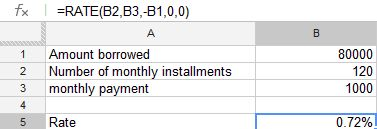the formula in cell B5 is =RATE(B2,B3,-B1,0,0)

I got the as 0.007241020100393 which i formatted using the % to convert it 0.72%

the above rate is period rate we have to convert this into annual rate

to convert this to annual rate we have multiplied it with 12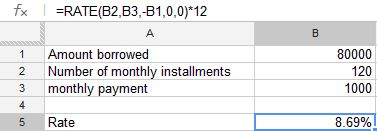for the formula in cell B5 is =RATE(B2,B3,-B1,0,0)*12

the solution to the problem is

i can afford up to 8.69% interest for my loan of 80,000/- for a period of 10 yearsThere is no function called Averageif in google docs spreadsheet as on October 2012. The average function return the arithmetic mean of a given data. If you want to eliminate certain value in the data and calculate the average of the remaining number the averageif comes into picture. We will using the average function only but we can manipulate in such a way to get the desired results

Example 1: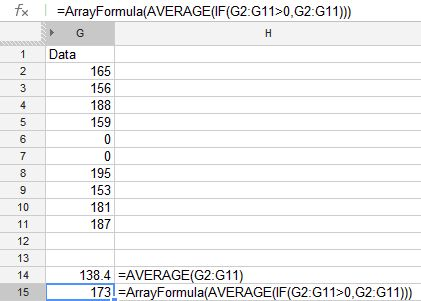The data is from Cell G2 to G11 containing 10 number if we want to calculate the average of numbers the formula will be =AVERAGE(G2:G11) which is equal to 138.4.

Suppose if we want to eliminate 0 (ZERO) while calculating the average then the formula will be

=ARRAYFORMULA(AVERAGE(IF(G2:G11>0;G2:G11)))

the result will be 173 as shown the above image.

Example 2: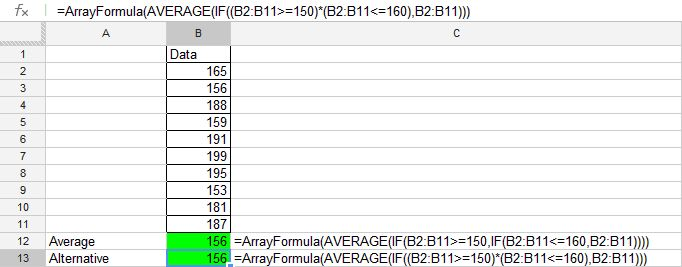in the above data set we want to calculate the average of the number which are between 150 and 160.

to achieve this we have used the following formula in cell B12

=ArrayFormula(AVERAGE(IF(B2:B11>=150;IF(B2:B11<=160;B2:B11))))

similarly the alternatives will be

=ArrayFormula(AVERAGE(IF((B2:B11>=150)*(B2:B11<=160);B2:B11)))

=AVERAGE(FILTER(B2:B11;(B2:B11>=150)*(B2:B11<=160)))

or

=AVERAGE(QUERY(B2:B11;”select B where B >=150 and B <=160″))

the above formula uses single IF statement whereas the previous formula uses the nested IF function which sometimes may become complicated to understand.

Similarly we can add any number of multiple conditions to calculate the average.

## Weighted Average or (Weighted Mean):

An Arithmetic Average that takes into account the importance of the items making up the average. In calculating the value of a share index, the share price are usually weighted in the some way, most often by the market capitalization of the company.

In Google Docs Spreadsheets Average function return the average of a range of data. if you want to calculate a weighted average there is no direct function to calculate this,  so we have to manipulate this using the some the native function available in google native functions.

Example:

Suppose a trader buys commodity on four occasions -100 tonnes at 70 per tonne, 300  tonnes at 80 per tonne, 50 tonnes at 95 per tonne and 60 tonnes at 75 per tonne. the purchase total 510 tonnes. the Simple average price would be (70+80+50+60)/4=65. However, the weighted average, taking into account the amount purchased on each transaction is

[(100 X 70) + (300 X 80) + (50 X 95) + (60 X 75)]/510=78.92

In the Spreadsheet this can be done using Sumproduct function and Sum function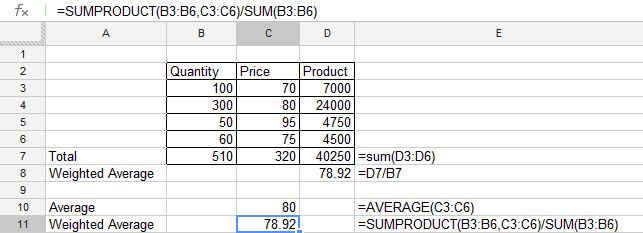in cell D8 we have used the basic formula as show the above example.

to get the single cell formula we have used the Sumproduct and sum formula to get the weighted average as show in the Cell C11.

In Cell C10 we have average formula